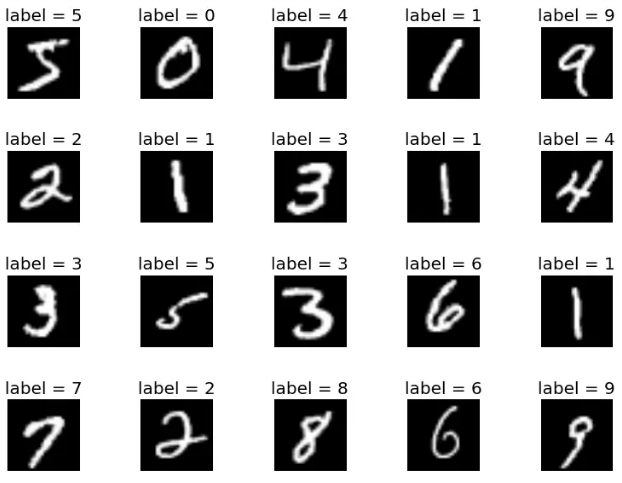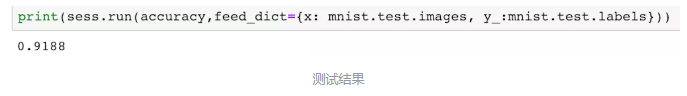## 机器学习实战—手写体数字识别（基于MNIST）

MNIST是非常有名的手写体数字识别数据集，在Tensorflow的官方网站里，第一个就拿它来做实战讲解，咱们也以此作为开始的项目。

MNIST（官方网站）是非常有名的手写体数字识别数据集，在Tensorflow的官方网站里，第一个就拿它来做实战讲解，咱们也以此作为开始的项目。它由手写体数字的图片和相对应的标签组成，如：MNIST数据集分为训练图像和测试图像。训练图像60000张，测试图像10000张，每一个图片代表0-9中的一个数字，且图片大小均为28*28的矩阵。

train-images-idx3-ubyte.gz: training set images (9912422 bytes) 训练图片

train-labels-idx1-ubyte.gz: training set labels (28881 bytes) 训练标签

t10k-images-idx3-ubyte.gz: test set images (1648877 bytes) 测试图片

t10k-labels-idx1-ubyte.gz: test set labels (4542 bytes) 测试标签

`source activate <virtual env> # virtual env是你的环境名字 `

`jupyter notebook `

```import tensorflow as tf

from tensorflow.examples.tutorials.mnist import input_data

```x = tf.placeholder(tf.float32, [None, 784])
y_ = tf.placeholder(tf.float32, [None, 10]) ```

```# W是模型的参数，是一个784*10的矩阵将一个784维的输入转换为一个10维的输出
W = tf.Variable(tf.zeros([784, 10]))
# b是偏置项（bias）。
b = tf.Variable(tf.zeros())
# y=softmax(Wx + b)，y表示模型的输出
y = tf.nn.softmax(tf.matmul(x, W) + b) ```

softmax是个简单的分类器，其结果是一个矩阵，分别代表着模型对于输入数据的预测属于各个分类的可能性。比如
[0.00, 0.01, 0.02, 0.01, 0.98, 0.01, 0.01, 0.00, 0.01, 0.02]

`cross_entropy = tf.reduce_mean(-tf.reduce_sum(y_ * tf.log(y))) `

`train_step = tf.train.GradientDescentOptimizer(0.01).minimize(cross_entropy) `

```sess = tf.InteractiveSession() # 创建一个Session
tf.global_variables_initializer().run() # 初始化所有变量，分配内存。 ```

```# 分1000步梯度下降
for _ in range(1000):
batch_x, batch_y = mnist.train.next_batch(100)
sess.run(train_step, feed_dict={x: batch_x, y_: batch_y}) ```

```# 正确的预测结果
correct_prediction = tf.equal(tf.argmax(y, 1), tf.argmax(y_, 1))
# 计算预测准确率
accuracy = tf.reduce_mean(tf.cast(correct_prediction, tf.float32))
# 传入测试数据集，获取模型测试的正确率
print(sess.run(accuracy, feed_dict={x: mnist.test.images, y_: mnist.test.labels})) ```

[0.00, 0.01, 0.02, 0.01, 0.98, 0.01, 0.01, 0.00, 0.01, 0.02]。所以我们使用tf.argmax(y, 1)之后得到的是最有可能的那个值，即4。
tf.equal返回的是boolean值，所以我们使用tf.cast将其转换成float便于计算。• 1888
• 摘抄
• https://www.jianshu.com/p/a2c9d39d90c6